×#### Thank you for registering.

One of our academic counsellors will contact you within 1 working day.

Click to Chat

1800-1023-196

+91-120-4616500

CART 0

• 0

MY CART (5)

Use Coupon: CART20 and get 20% off on all online Study Material

ITEM
DETAILS
MRP
DISCOUNT
FINAL PRICE
Total Price: Rs.

There are no items in this cart.
Continue Shopping• Complete JEE Main/Advanced Course and Test Series
• OFFERED PRICE: Rs. 15,900
• View Details

```Congruence Exercise 16.3

Question: 1

By applying SAS congruence condition, state which of the following pairs of triangle are congruent. State the result in symbolic form

Solution:

(i)We have OA = OC and OB = OD and

∠AOB = ∠COD which are vertically opposite angles. Therefore by SAS condition, ΔAOC ≅ ΔBOD

(ii)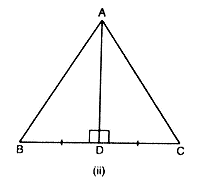We have BD = DC

(iii)We have AB = DC

∠ABD = ∠CDB and

Therefore, by SAS condition, ΔABD ≅ ΔCBD

(iv)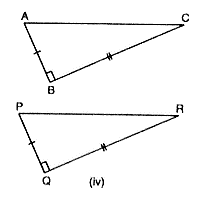We have BC = QR

ABC = PQR = 90°

And AB = PQ

Therefore, by SAS condition, ΔABC≅ ΔPQR.

Question: 2

State the condition by which the following pairs of triangles are congruent.

Solution:

(i)BC = CD and AC = CA

Therefore by SSS condition, ΔABC≅ ΔADC

(ii)AC = BD

AD = BC and AB = BA

Therefore, by SSS condition, ΔABD ≅ ΔADC

(iii)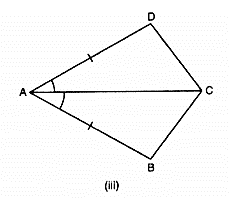∠BAC = ∠DAC and

Therefore by SAS condition, ΔBAC ≅ ΔBAC

(iv)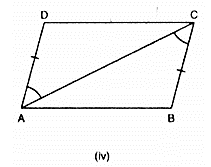∠DAC = ∠BCA and

Therefore, by SAS condition, ΔABC ≅ ΔADC

Question: 3

In figure, line segments AB and CD bisect each other at O. Which of the following statements is true?

(i) ΔAOC ≅ ΔDOB

(ii) ΔAOC ≅ ΔBOD

(iii) ΔAOC ≅ ΔODB

State the three pairs of matching parts, you have used to arrive at the answer.Solution:

We have,

And, CO = OD

Also, AOC = BOD

Therefore, by SAS condition, ΔAOC ≅ ΔBOD

Question: 4

Line-segments AB and CD bisect each other at O. AC and BD are joined forming triangles AOC and BOD. State the three equality relations between the parts of the two triangles that are given or otherwise known. Are the two triangles congruent? State in symbolic form, which congruence condition do you use?

Solution:

We have AO = OB and CO = OD since AB and CD bisect each other at 0.

Also ∠AOC = ∠BOD since they are opposite angles on the same vertex.

Therefore by SAS congruence condition, ΔAOC ≅ ΔBOD

Question: 5

ΔABC is isosceles with AB = AC. Line segment AD bisects ∠A and meets the base BC in D.

(ii) State the three pairs of matching parts used to answer (i).

(iii) Is it true to say that BD = DC?

Solution:

(i) We have AB = AC (Given)

Therefore by SAS condition of congruence, ΔABD ≅ ΔACD

(iii) Now, ΔABD≅ΔACD therefore by c.p.c.t BD = DC.

Question: 6

In Figure, AB = AD and ∠BAC = ∠DAC.

(i) State in symbolic form the congruence of two triangles ABC and ADC that is true.

(ii) Complete each of the following, so as to make it true:

(a) ∠ABC =

(b) ∠ACD =

(c) Line segment AC bisects ___ and ___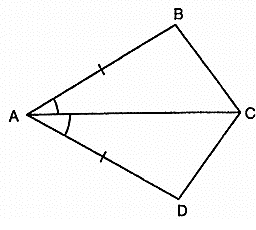Solution:

∠BAC = ∠DAC (given)

AC = CA (common)

Therefore by SAS condition of congruency, ΔABC ≅ ΔADC

∠ACD = ∠ACB (c.p.c.t)

Question: 7

In figure, AB || DC and AB = DC.

(i) Is ΔACD ≅ ΔCAB?

(ii) State the three pairs of matching parts used to answer (i).

(iii) Which angle is equal to ∠CAD ?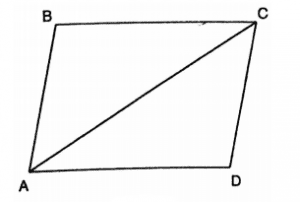Solution:

(i) Yes by SAS condition of congruency, ΔDCA ≅ ΔBAC

(ii) We have used AB = DC, AC = CA and ∠DCA = ∠BAC.

(iii) ∠CAD = ∠ACB since the two triangles are congruent.

(iv) Yes this follows from AD // BC as alternate angles are equal. lf alternate angles are equal the lines are parallel
```### Course Features

• 728 Video Lectures
• Revision Notes
• Previous Year Papers
• Mind Map
• Study Planner
• NCERT Solutions
• Discussion Forum
• Test paper with Video Solution